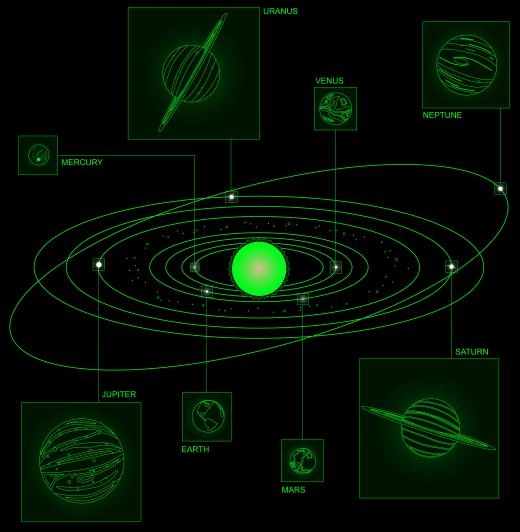Science
Fact Checked

# What Is the Law of Universal Gravitation?

Alan Rankin
Alan Rankin

The law of universal gravitation is an essential principle of physics. It was first codified by Sir Isaac Newton in the 1600s. It states that all objects are attracted to each other by gravity; the force of the attraction depends on the mass of the objects and decreases based on the distance between them. Newton’s discovery was superseded by Einstein’s theory of general relativity. It is still accurate, however, for most practical applications.

Newton did not discover gravity, as the popular belief holds, but expanded on the work of earlier scientists such as Galileo. Newton referred to these scientists when he famously wrote, “If I have seen further, it is by standing on the shoulders of giants.” The fall of an apple inspired Newton to study the subject of gravity; however, the apple did not bring instant understanding by hitting his head. Instead, he used the moon’s orbit around the Earth to check and confirm his calculations over a span of 20 years. The law of universal gravitation was detailed in his groundbreaking book Principia Mathematica, published in 1687.

Newton’s book included mathematical formulas describing the law of universal gravitation. In essence, the law states that all objects exert a gravitational pull on all other objects. Objects with a great deal of mass have stronger areas of gravity, or gravitational fields, which is why objects and people are attracted to the Earth, but not noticeably to each other. The gravitational pull decreases as distance increases; this decrease can be precisely measured, and is known in physics as an inverse-square law. Universal gravitation is the force that keeps planets and satellites locked in orbit, rather than traveling freely about the universe.The Sun's gravitational pull keeps the planets of the Solar System orbiting around it.

In the centuries after Newton’s lifetime, the law of universal gravitation was used to predict the locations of planets and natural satellites that had not yet been discovered. The eventual discoveries of these celestial bodies confirmed that the law was correct. One aspect of the law Newton could not explain was how gravitational force is conveyed between objects. Other fundamental forces, such as electromagnetism, work because sub-atomic particles travel between the objects, attracting them to each other. A similar particle for conveying gravity, the graviton, has been described in theory, but remains undiscovered more than 300 years after Newton’s work.

By the 20th century, scientists had discovered minor inconsistencies in the law of universal gravitation. These inconsistencies were explained by Einstein’s theory of general relativity. Einstein realized that, in effect, the masses of celestial bodies not only affect each other, but the fabric of space-time around them. These effects are noticeable only in very precise measurements and calculations. For practical applications such as rocket launches, the law of universal gravitation is still accurate and much easier to calculate than the effects of relativity.

## You might also Like